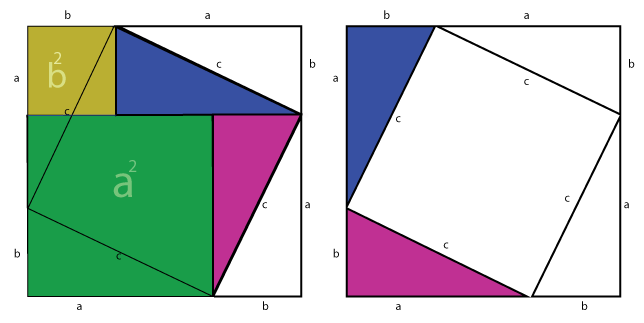Can you describe the proof by using the following pictures?Hint: A key point is: If you cut the blue and pink triangles and paste them to the position shown in the left picture above, you get a square with area $$c^2$$.

Here is a link for 100 proofs of Pythagorean Theorem:

Also 3-d Pythagorean Theorem will be trivial to prove, but can you describe and generalize it?